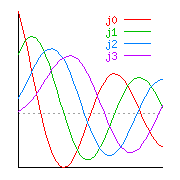SoftIntegration Solutions
 Middle and High Schools  Colleges and Universities  Enterprise  Embedded Scripting  Plotting/Numerical Computing
Solutions for Scientific Computing in Engineering and Science
Ch is the simplest possible solution to
2D/3D plotting and numerical computing
within the framework of C/C++

There are numerous problems in engineering and science that have to be solved with scientific numerical computing and visualization. Ch is an ideal computing environment for engineers and scientists to solve their challenging problems productively. Ch contains salient features with simplicity of Fortran and mathematical software packages such as MATLAB® and Mathematica® to ease engineering and scientific numerical computing.

If you are familiar with mathematical software packages MATLAB® and Mathematica®, you may find the following information helpful in understanding the capabilities of Ch.Ch is a congenial development environment for numerical programming. I can certainly recommend Ch to scientists, engineers and C/C++ programmers." -- Dr. Martin Heller, Mr. Computer Language Person at BYTE.comCh has been developed to meet most computing needs for engineers and scientists. Engineers and scientists select Ch as their solution for the following reasons:
• Power
As a superset of C with classes in C++, Ch has a very rich set of features for scientific numerical computing and visualization, including all new features for numerical computing in C99 such as IEEE floating-point arithmetic, complex numbers, variable length arrays (VLAs), and generic functions. Ch is the only computing environment in existence that can perform numerical computing with consistent numerical results under the IEEE floating-point arithmetic in the entire real domain and complex domain using an extended complex plane for a Riemman sphere.

Unlike special mathematical software packages, Ch conforms to many industry standards. For example, wide characters is supported in Ch for internationalization. You can develop scientific numerical computing applications with LAPACK, 2D/3D plotting, CGI, OpenGL, ODBC, GTK+, X-Windows and Motif.

• Cost saving
Prior to Ch, one may use C/C++ for general algorithm development and graphical packages or Excel for display and visualization; or use expensive mathematical software packages such as MATLAB and Mathematica for rapid prototyping, then generate C/C++ code for final production. Any change to machine geneated C/C++ code has to be propagated from the code written in MATLAB or Mathematica. With Ch, all development can be accomplished in a single language environment. This not only reduces the cost for purchase of multiple software packages and compilers from different vendors, but also the cost for development and maintanence of code with higher productivity.

• Efficiency and easy of use
Ch is a very high-level language (VHLL) environment. It can conveniently glue different applications and components together. Ch encourages code reuse. Thousands of lines of C/C++ code for numerical computing can be accomplished with only a few lines of Ch code. Application programs are easy to develop and maintain. Extensive numerical features with advanced library and graphical plotting dramatically simplify algorithm development and visualization. Computational arrays allow matrices to be manipulated as first-class objects so that mathematical formulas such as linear equation b = A*x can be written verbatim in Ch. The user does not need to worry about the underlying optimization with fast and accurate numerical algorithms.

Ch is especially suitable for development of Web-based applications in engineering and science as shown in online scientific numerical computing and visualization.

• Third Party Solutions
An increasing number of Ch toolkits and third party packages are supported. Ch Control Toolkit, Ch NAG Statistics Package, Ch OpenCV package, etc. make Ch a powerful platform for engineers and scientists.
Note: To compile graphical functions with your applications, please try our SoftIntegration C++ Graphic al Library (SIGL).